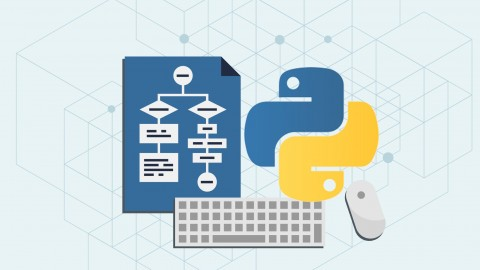# UdemyAlgorithms and Data Structures in PythonWhat you'll learn
• Understand arrays and linked lists
• Understand stacks and queues
• Understand the differences between data structures and abstract data types
• Understand tree like data structures (binary search trees)
• Understand balances trees (AVL trees and red-black trees)
• Understand graph traversing (BFS and DFS)
• Understand shortest path algorithms such as Dijkstra's approach
• Understand minimum spanning trees
• Be able to develop your own algorithms
• Have a good grasp of algorithmic thinking
• Be able to detect and correct inefficient code snippets
Requirements
• Python basics
• Some theoretical background ( big O notation )
Description
This course is about data structures, algorithms and graphs. We are going to implement the problems in Python programming language. I highly recommend typing out these data structures and algorithms several times on your own in order to get a good grasp of it.
So what are you going to learn in this course?
Section 1:
• setting up the environment
• differences between data structures and abstract data types
Section 2:
• what is an array data structure
• arrays related interview questions
• linked list data structure and its implementation
Section 3:
• stacks and queues
• stacks and queues related interview questions
Section 4:
• what are binary search trees
• practical applications of binary search trees
Section 5:
• problems with binary trees
• balanced trees: AVL trees and red-black trees
Section 6:
• what are heaps
• heapsort algorithm overview
Section 7:
• associative arrays and dictionaries
• how to achieve O(1) constant running time with hashing
• ternary search trees as associative arrays
Section 8:
• basic graph algorithms
Section 9:
• shortest path algorithms
• Dijkstra's algroithm
• Bellman-Ford algorithm
Section 10:
• what are spanning trees
• what is the union-find data structure and how to use it
• Kruskal's algorithm theory and implementation as well
Section 11:
• sorting algorithms
• bubble sort, selection sort and insertion sort
• quicksort and merge sort
• non-comparison based sorting algorithms
• counting sort and radix sort
In the first part of the course we are going to learn about basic data structures such as linked lists, stacks, queues, binary search trees, heaps and some advanced ones such as AVL trees and red-black trees.. The second part will be about graph algorithms such as spanning trees, shortest path algorithms and graph traversing. We will try to optimize each data structure as much as possible.
In each chapter I am going to talk about the theoretical background of each algorithm or data structure, then we are going to write the code step by step in Python.
Most of the advanced algorithms relies heavily on these topics so it is definitely worth understanding the basics. These principles can be used in several fields: in investment banking, artificial intelligence or electronic trading algorithms on the stock market. Research institutes use Python as a programming language in the main: there are a lot of library available for the public from machine learning to complex networks.
Thanks for joining the course, let's get started!
Who this course is for:
• Beginner Python developers curious about graphs, algorithms and data structures
•••thorpham, Pulu, anhtung and 48 others
Author
jpyo004